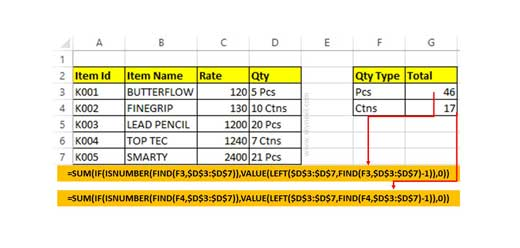# How To Sum Cells With Text And Numbers appended in same cell in Excel?

Normally you can't do this job, but Sum array allows you to get the get the sum cells with text & numbers appended in same cell Excel Sheet.

During working on Excel, sometime we have a list of values which containing numerical along with textual values both in a single cell. To get total or count these types of cells very difficult. In this guide we have to discuss how to sum cells with text and numbers appended in same cell in Excel. Normally, when working on Excel sheet you can’t able to apply sum function on that type of values, which have both numerical and text string. In that situation you have to do this job manually, which is very time consuming. So, you have to use given function which helps you to sum cells with text and numbers in excel sheet.

Excel allows you to use an array formula to quickly get the sum of those cells in which have both text string and numerical value. If you doing this job with normal SUM function with simple range of cells then you can’t get correct result. So, in that situation you must have to use array sum function which allows you to get the correct result.

### 5 Ways to use SUM Function in MS Excel

When you are working in a large worksheet data and you need to get the total of numbers of particular range of cells and want to save your time. Microsoft Excel, provide the different ways from which you can quickly calculate total numbers in columns and row by using SUM function in MS Excel. It’s a very popular math and trigonometry function.

The Math functions carry out all the basic mathematical task such as add, subtract, multiply and divide any numbers, while the trig functions are used to calculate the result of the sine, cos, and tan and other thetas of an angle as well as converting angle sizes between the degrees. The SUM function is most popular function of Math and Trig. Functions category in MS Excel.

## Steps to Sum Cells With Text And Numbers appended in same cell in Excel

For e.g. If you have a worksheet which have both numerical and text values. Now, you want to get sum which matched with text string. In that situation, you must have to use SUM array function to get the correct result.Step 1: Open the worksheet in which you have both numerical and text values in a single or range of cells.

Step 2: Type the given array function =SUM(IF(ISNUMBER(FIND(F3,\$D\$3:\$D\$7)),VALUE(LEFT(\$D\$3:\$D\$7,FIND(F3,\$D\$3:\$D\$7)-1)),0)). Now, press Ctrl + Shift + Enter shortcut keys together, at the place of hit enter key. After that you will get the total value from the list which is matched with the given text string.

Now, drag the given function for rest of cells. It will help’s you to get the total of numerical values which matched with text string.

### How to use VLookup and Sum function to get total for Multiple columns

Most of the person want to become an Excel expert and they need more practice on different functions. In this guide we have to discuss how to use VLookup and SUM function to get the total for multiple columns in Excel sheet. During preparing the MIS reports you must have to use VLookup function to search the specific value.

During working on a large worksheet or an array you have to search and get the total of all those values values which meet the condition you specify in the same or other worksheet. In that situation you must have to get proper knowledge of advanced VLookup
function which allows you to get the result of your query.